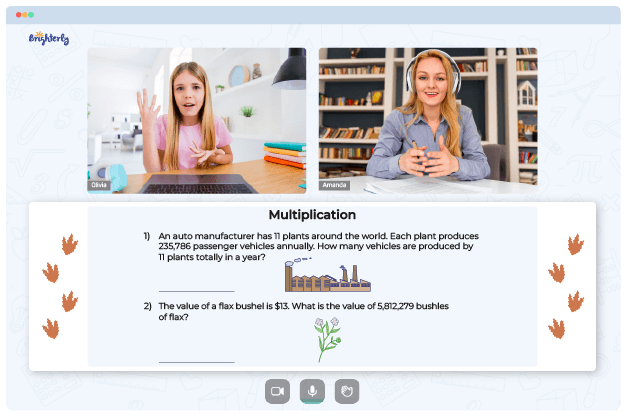# Multiplying And Dividing Integers Worksheets

A multiply and dividing integers worksheets for graders help them to grasp the rationale behind these operations. Integer multiplication and division are foundational mathematical concepts that will stay with the learner as they progress through school.

## Free multiplying and dividing integers worksheets

Using free multiplying and dividing integers worksheets can boost kids’ problem-solving abilities and self-confidence.

If used by 7th graders, multiplying and dividing integers word problems worksheets cover vital topics such as finding the products of two integers, principles of multiplying or dividing integers, applying integers, and finding the quotient after dividing two integers.

Continued practice with worksheets may improve time management skills of students, which can be helpful in exams and assessments. Teachers may exploit this incredible perk of multiplying and dividing integers worksheets to challenge kids to compete with their peers, using time spent and score as yardsticks.

Math for Kids

Is Your Child Struggling With Math?
1:1 Online Math TutoringGetting a multiply and divide integers worksheet will help your kids become excellent in other mathematical concepts. As kids practice with worksheets, their self-confidence and critical thinking will improve significantly; hence, fear of mathematics will be removed.

The stepwise approach used in the design of worksheets ensures that kids will not feel pressured while learning. They will start from relatively simple to complex topics, which allows them to learn at their own pace.

## Printable multiplying and dividing integers worksheet PDF### Multiplying And Dividing Integers Worksheets PDF

Multiplying And Dividing Integers Worksheet### Multiplying And Dividing Integers Worksheets PDF

Multiply And Dividing Integers Worksheets### Multiplying And Dividing Integers Worksheets PDF

Multiplying And Dividing Integers Worksheet PDF### Multiplying And Dividing Integers Worksheets PDF

Multiplying And Dividing Integers Worksheets

You don’t need to use your computer whenever you want your kids to practice with the integer worksheet. Having the printable version available on Brighterly will help you keep your kids busy and teach them to learn independently.

With a paper worksheet, your kid can repeatedly practice without supervision. This continued practice leads to mastery of the concept.

### More Integers Worksheets

Need help with Multiplication?• Is your child finding it challenging to understand multiplication?
• An online tutor could be the solution.

Does your child struggle to master the concept of multiplication? Try studying with an online tutor.